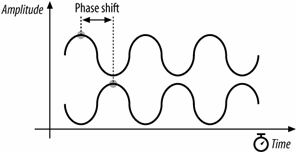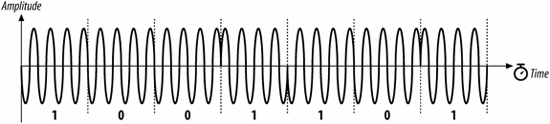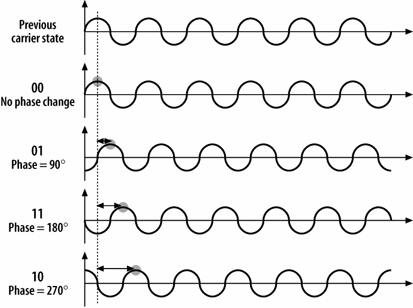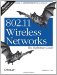# Differential Phase Shift Keying (DPSK)

Differential phase shift keying (DPSK) is the basis for 802.11 direct-sequence systems. As the name implies, phase shift keying (PSK) encodes data in phase changes of the transmitted signal. The absolute phase of a waveform is not relevant in PSK; only changes in the phase encode data. Like frequency shift keying, PSK resists interference because most interference causes changes in amplitude. Figure 12-8 shows two identical sine waves shifted by a small amount along the time axis. The offset between the same point on two waves is the phase difference.

Figure 12-9. Phase difference between two sine wavesDifferential Binary Phase Shift Keying (DBPSK)

The simplest form of PSK uses two carrier waves, shifted by a half cycle relative to each other. One wave, the reference wave, is used to encode a 0; the half-cycle shifted wave is used to encode a 1. Table 12-2 summarizes the phase shifts.

Table 12-2. DBPSK phase shifts

Symbol

Phase shift

0

0

1

Figure 12-10 illustrates the encoding as a phase difference from a preceding sine wave.

Figure 12-10. DBPSK encodingTo stick with the same example, encoding the letter M (1001101 in binary) is a matter of dividing up the time into seven symbol times then transmitting the wave with appropriate phase shift at each symbol boundary. Figure 12-11 illustrates the encoding. Time is divided into a series of symbol periods, each of which is several times the period of the carrier wave. When the symbol is a 0, there is no change from the phase of the previous symbol, and when the symbol is a 1, there is a change of half a cycle. These changes result in "pinches" of the carrier when 1 is transmitted and a smooth transition across the symbol time boundary for 0.

Figure 12-11. The letter M encoded in DBPSKDifferential Quadrature Phase Shift Keying (DQPSK)

Like 2GFSK, DBPSK is limited to one bit per symbol. More advanced receivers and transmitters can encode multiple bits per symbol using a technique called differential quadrature phase shift keying (DQPSK). Rather than a fundamental wave and a half-cycle shifted wave, DQPSK uses a fundamental wave and three additional waves, each shifted by a quarter cycle, as shown in Figure 12-12. Table 12-3 summarizes the phase shifts.

Figure 12-12. DQPSK encodingTable 12-3. DQPSK phase shifts

Symbol

Phase shift

00

0

01

11

10

Now encode M in DQPSK (Figure 12-13). In the UTF-8 character set, M is represented by the binary string 01001101 or, as the sequence of four two-bit symbols, 01-00-11-01. In the first symbol period, there is a phase shift of 90 degrees; for clarity, the figure shows the phase shift from a pure sine wave. The second symbol results in no phase shift, so the wave continues without a change. The third symbol causes a phase shift of 180 degrees, as shown by the sharp change from the highest amplitude to the lowest amplitude. The final symbol causes a phase shift of 90 degrees.

Figure 12-13. The letter M encoded in DQPSKThe obvious advantage of DQPSK relative to DBPSK is that the four-level encoding mechanism can have a higher throughput. The cost of using DQPSK is that it cannot be used in some environments because of severe multipath interference. Multipath interference occurs when the signal takes several paths from the transmitter to the receiver. Each path has a different length; therefore, the received signal from each path has a different delay relative to the other paths. This delay is the enemy of an encoding scheme based on phase shifts. Wavefronts are not labeled or painted different colors, so a wavefront could arrive later than expected because of a long path or it could simply have been transmitted late and phase-shifted. In environments where multipath interference is severe, DQPSK will break down much quicker than DBPSK.802.11 Wireless Networks: The Definitive Guide, Second Edition
ISBN: 0596100523
EAN: 2147483647
Year: 2003
Pages: 179
Authors: Matthew Gast